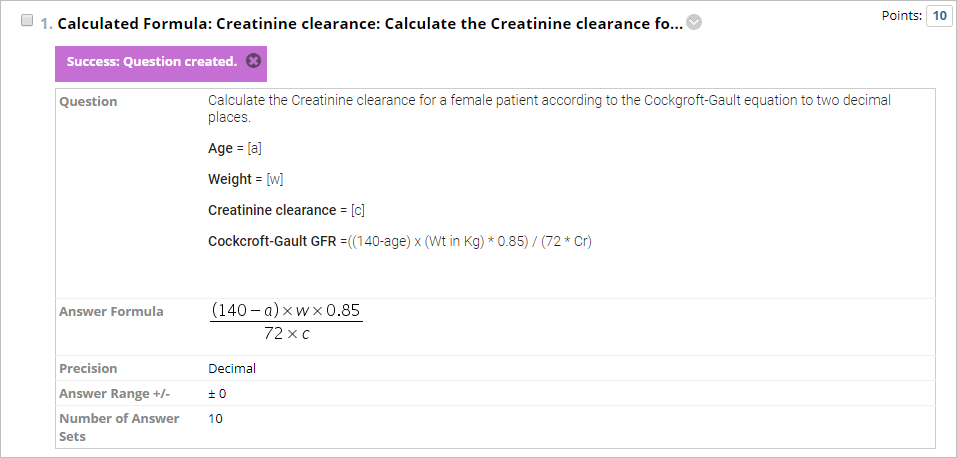## Video Guide

Create a Calculated Formula Question (YouTube, 3m 1s)

## Overview

Questions can be added to a test (refer Create a Test guide) or pool (refer Create a Pool guide).

A Calculated Formula question contains a formula, the variables of which are set to change for each student. You specify the minimum and maximum value for each variable and answer sets are randomly generated.

## Example question

 Calculate the Creatinine clearance for a female patient according to the Cockgroft-Gault equation to two decimal places. Age = ? Weight = ? Creatinine clearance = ? Cockcroft-Gault GFR = ((140-age) x (Wt in Kg) * 0.85) / (72 * Cr)

### Select question type

1. Click on the Create Question button.
2. Select Calculated Formula from the dropdown list.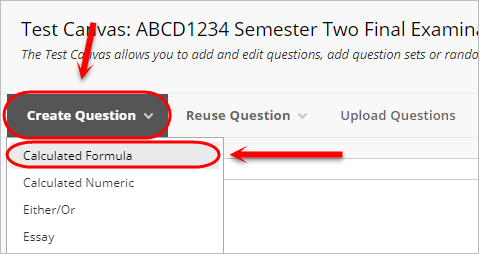### Question

1. Enter a title for the question in the Question Title textbox.
2. Enter the question in the Question Text textbox.
3. Include unique variables in square brackets (e and pi cannot be used as variables).

Note: A variable is not required for the answer.

1. Optionally, to add an image within the Question Text textbox, refer to the Insert an Image using the Text Editor guide.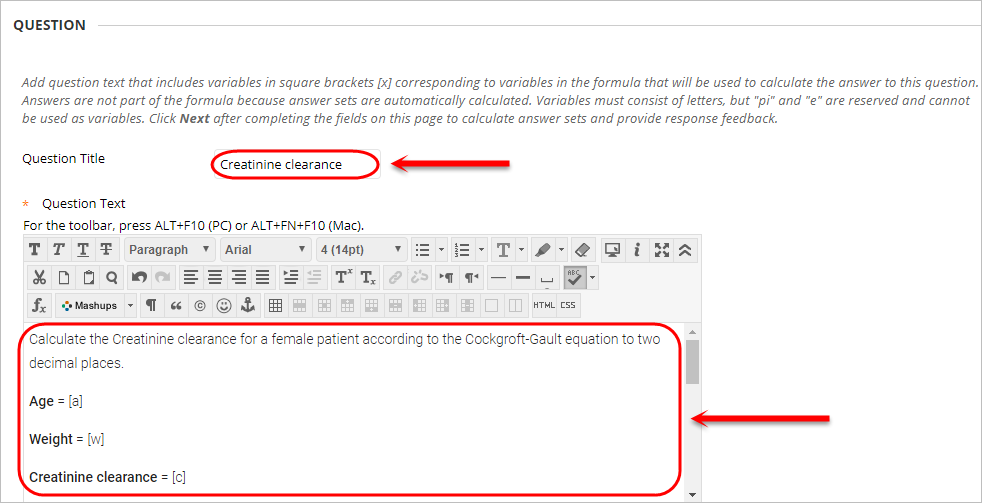1. Enter the required formula in the equation editor using the variables from the question.

Note: A variable for the answer and an equal sign are not required.

Warning: You must use the equation editor buttons to create the formula (i.e. click on the button instead of entering "x" into the textbox).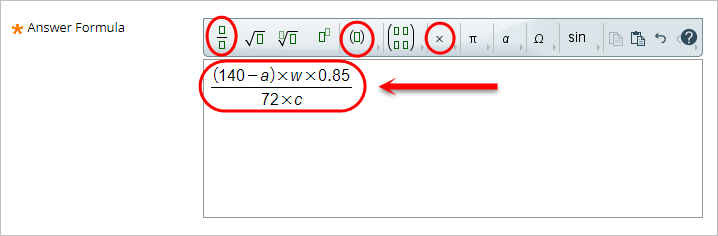### Options

1. Enter the accuracy range for a correct answer in Answer Range +/- textbox.

Note:  This option allows you to specify one correct answer (i.e. Answer Range = 0) or a range of answers.

1. Optionally, to award partial credit, check the Allow Partial Credit checkbox.
• Enter the accuracy range into the Partial Credit Range +/- textbox.
• Enter percentage into the Partial Credit Points Percentage textbox.

Note:  This option could be used to give partial credit for rounding errors (i.e. if the answer is to 2 decimal places, set the Partial Credit Range to 0.01).

1. Click on the Next Button.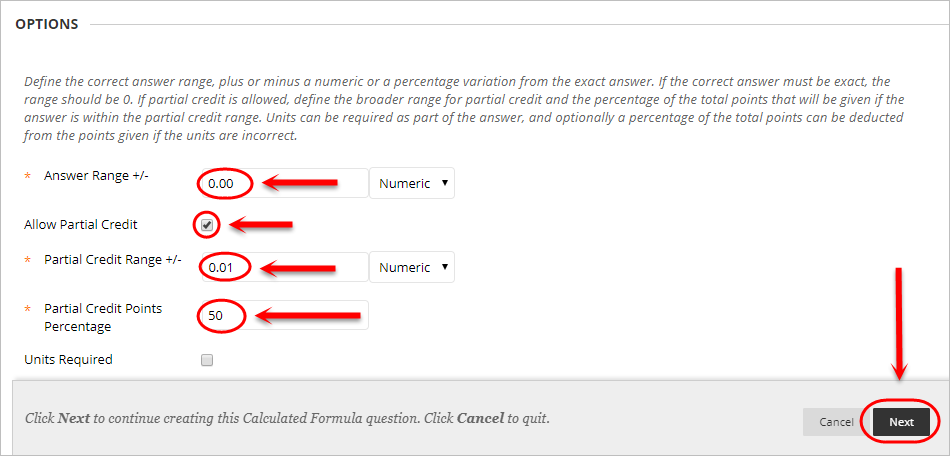### Define Variables

1. For each variable:

• Enter the lowest value possible in the Minimum Value textbox.
• Enter the highest value possible in the Maximum Value textbox.
• Select the required number of decimal places from the Decimal Places dropdown list.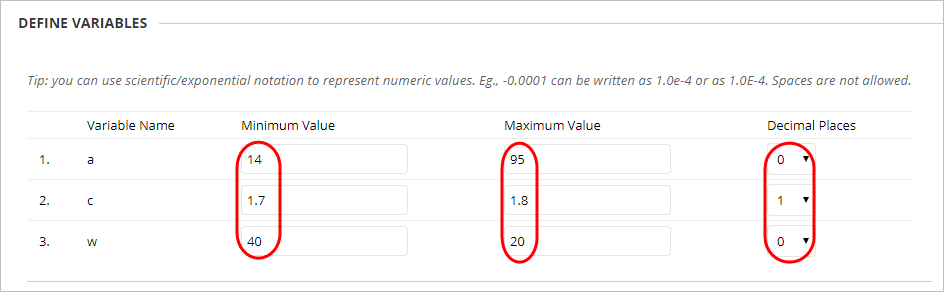1. Select the required number of decimal places from the Decimal Places for Answer dropdown list.
2. Enter the required number of answer sets in the Number of Answer Sets textbox.
3. Select Normal or Exponential from the Correct Answer Format dropdown list.
4. Click on the Calculate button to view the answer sets.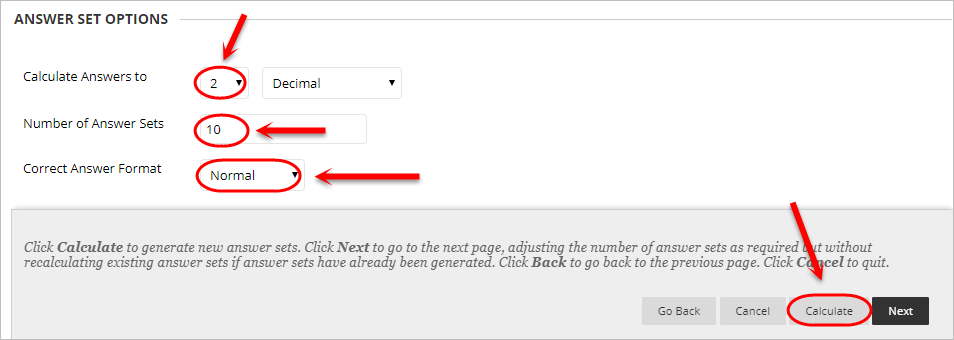1. Optionally, click on the Remove button to remove an answer set.

Tip: Remove any answer sets that are too easy compared to the others.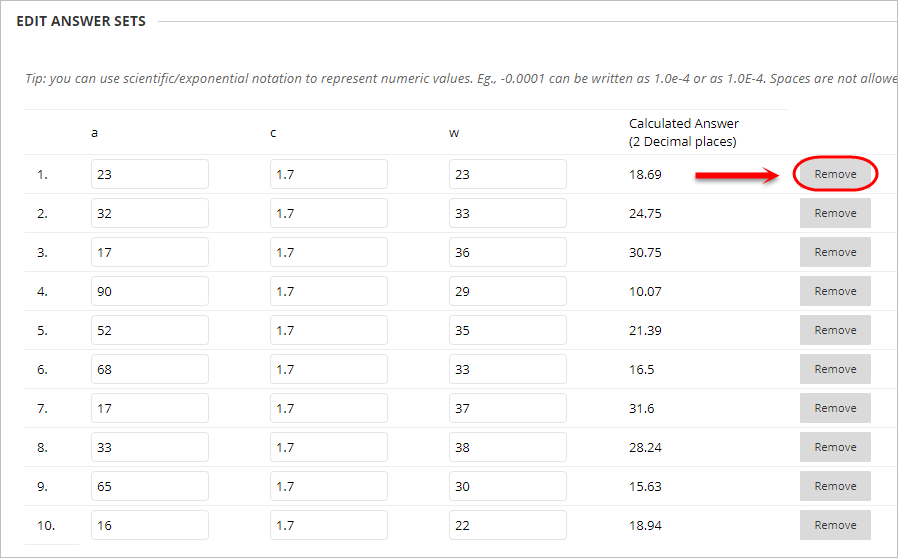### Feedback (optional)

Giving specific correct or incorrect response feedback is optional.

1. Enter correct response feedback in the Correct Response Feedback textbox.
2. Enter incorrect response feedback in the Incorrect Response Feedback textbox.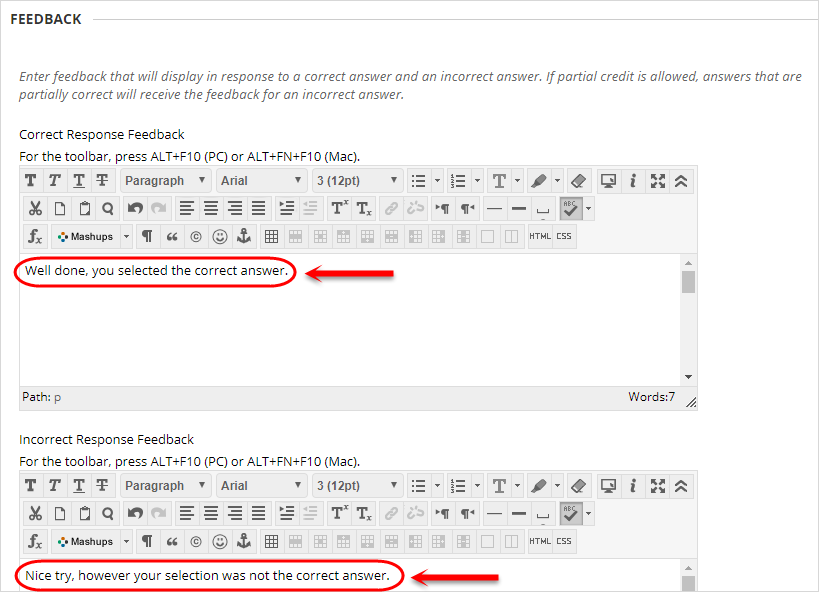### Categories and Keywords (optional)

Adding categories and keywords to questions allows you to search your tests and pools for questions by topic, category, level of difficulty or keyword.

Add a new topic/category/level of difficulty/keyword:

1. Click on the Add button.
2. Enter a word in the Add Tag textbox.
3. Click on the OK button to submit.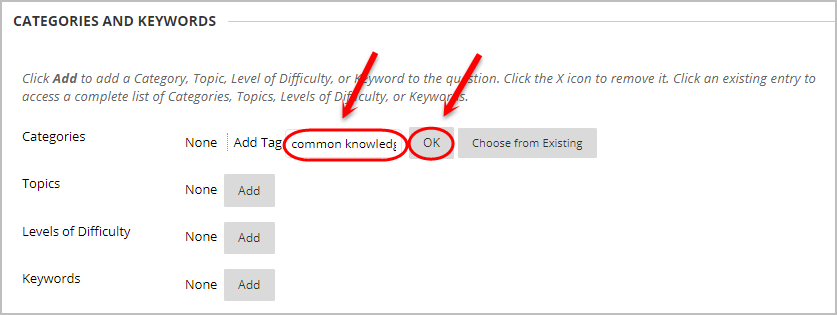Add an existing topic/category/level of difficulty/keyword:

1. Click on the Add button.
2. Click on the Choose from Existing button.
3. Select a word from the dropdown list.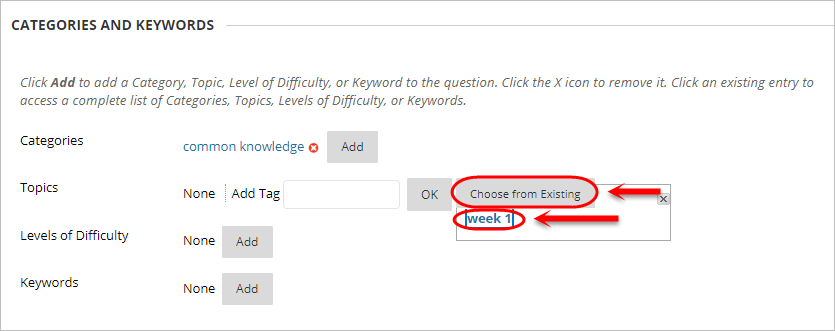Tip: Click on the (x) button to delete a topic/category/level of difficulty/keyword.

### Instructor Notes (optional)

1. Enter any notes for the marker in the Instructor Notes textbox.

Note: Instructor notes cannot be viewed by students.​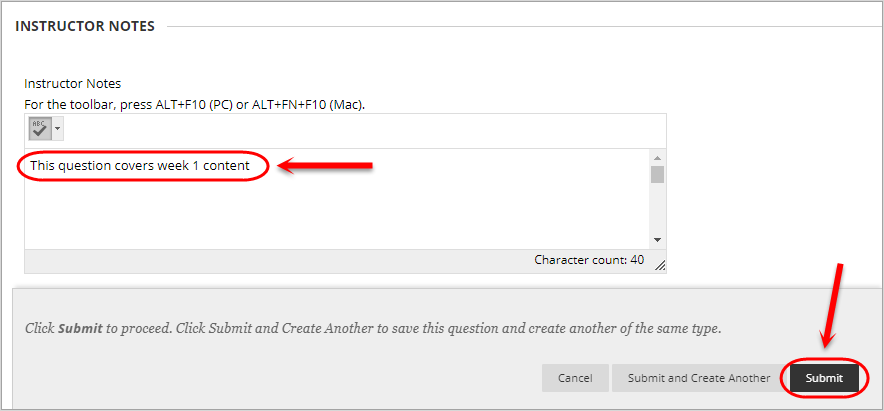### Submit

1. Click on the Submit button.